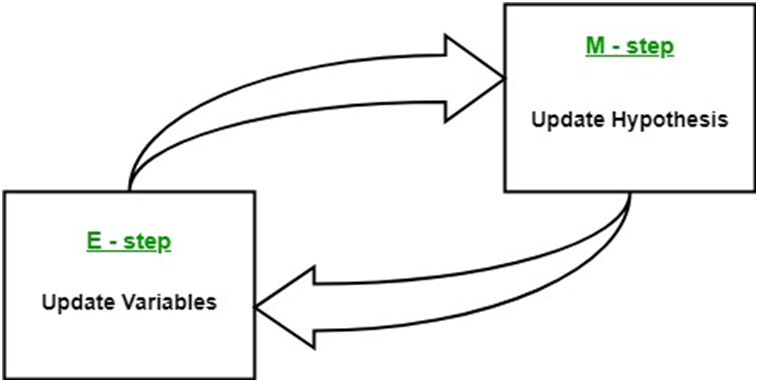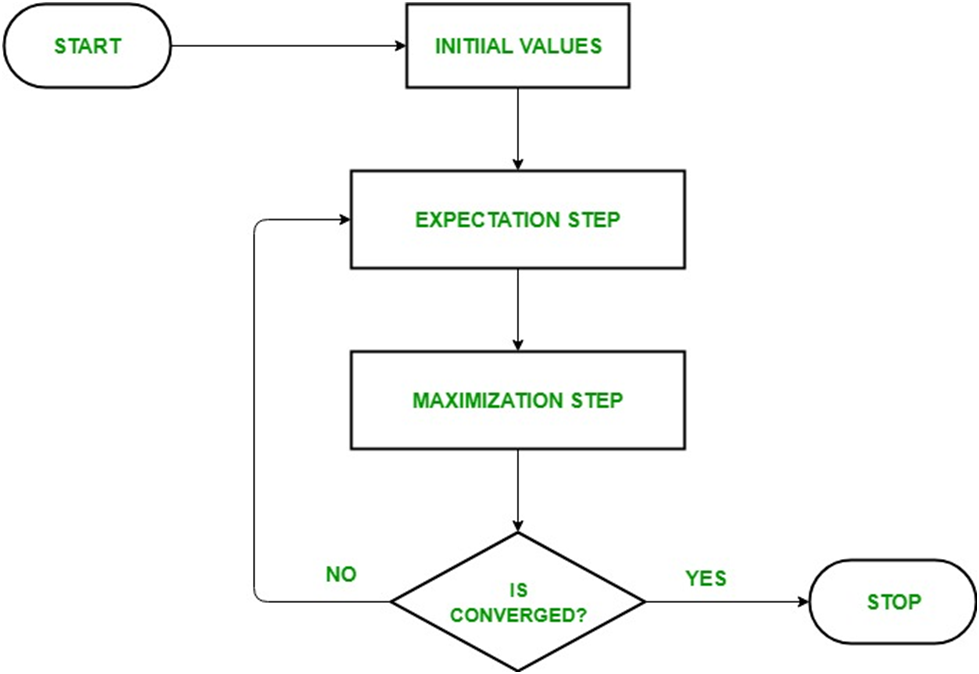### EM Algorithm

EM Algorithm

Algorithm:

• Given a set of incomplete data, consider a set of starting parameters.

• Expectation step (E – step): Using the observed available data of the dataset, estimate (guess) the values of the missing data.

• Maximization step (M – step): Complete data generated after the expectation (E) step is used in order to update the parameters.

• Repeat step 2 and step 3 until convergence.Usage of EM Algorithm

• It can be used to fill the missing data in a sample.

• It can be used as the basis of unsupervised learning of clusters.

• It can be used for the purpose of estimating the parameters of Hidden Markov Model (HMM).

• It can be used for discovering the values of latent variables.

• It is always guaranteed that likelihood will increase with each iteration.

• The E-step and M-step are often pretty easy for many problems in terms of implementation.

• Solutions to the M-steps often exist in the closed form.